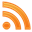# Sp4ce.net## Error detection in string with javascript

When you need to use the power of javascript in order to detect errors in strings. It detects only insertion and deletion of caracters in string.

### String comparison

When you want to compare string in order to detect errors, it can get very complicated. You can use a simple function like this

``````function compare(string1, string2, i, j) {
// We are a the end of one of the string, return the differences of caracters (= numbers of errors).
if (string1.length == i || string2.length == j) {
return Math.abs(string1.length - string2.length);
}

if (string1[i] == string2[j]) {
// The caracters are equal, we continue to the next caracters.
compare(string1, string2, i + 1, j + 1);
} else {
// We test BOTH insertion and deletion and return the least error weight.
var insertion = compare(string1, string2, i + 1, j);
var deletion = compare(string1, string2, i, j + 1);
return Math.min(insertion, deletion) + 1;
}
}
``````

However this have a bad complexity because it will compare again part of the string that has been already compare in another path of the comparison. For example, if you compare ‘abcdefgh’ with ‘aabcdefgh’, you go into a deep recursion.

By using a backtracking algorithm, we can reduce the complicity to be polynomial.

### Backtracking matrix

The main idea is to initialize the border of a matrix, because we know the value (the difference between the length), and then backtrack the values until the beginning of the strings.

 h e l l o h 4 l 3 l 2 o 1 5 4 3 2 1 0

The backtrack is very easy, you use this function:

``````function(reference, input, i, j) {
if (reference[i] == input[j])
matrix[i][j] = matrix[i + 1][j + 1];
else {
matrix[i][j] = Math.min(matrix[i + 1][j], matrix[i][j + 1]) + 1;
}
}
``````

And if you want to remember the path you made thought the backtracking, the matrix value became an object and the backtracking function will be:

``````function(reference, input, i, j) {
if (reference[i] == input[j])
matrix[i][j] = {
value: matrix[i + 1][j + 1].value,
direction: 'diagonal'
};
else if (matrix[i + 1][j].value < matrix[i][j + 1].value) {
matrix[i][j] = {
value: matrix[i + 1][j] + 1,
direction: 'down'
};
else {
matrix[i][j] = {
value: matrix[i][j + 1] + 1,
direction: 'right'
};
}
}
``````

And so the backtracking matrix becomes something that look like this. The path that shows the error is highlighted in red. It shows where it detects insertion or deletion.

 h e l l o h 1 2 1 2 3 4 l 2 1 0 1 2 3 l 3 2 1 0 1 2 o 4 3 2 1 0 1 5 4 3 2 1 0

You can find the entire source code and examples on github.
You can play with the working code here.

This work was done with the help of Jean-Marie Larchevêque.